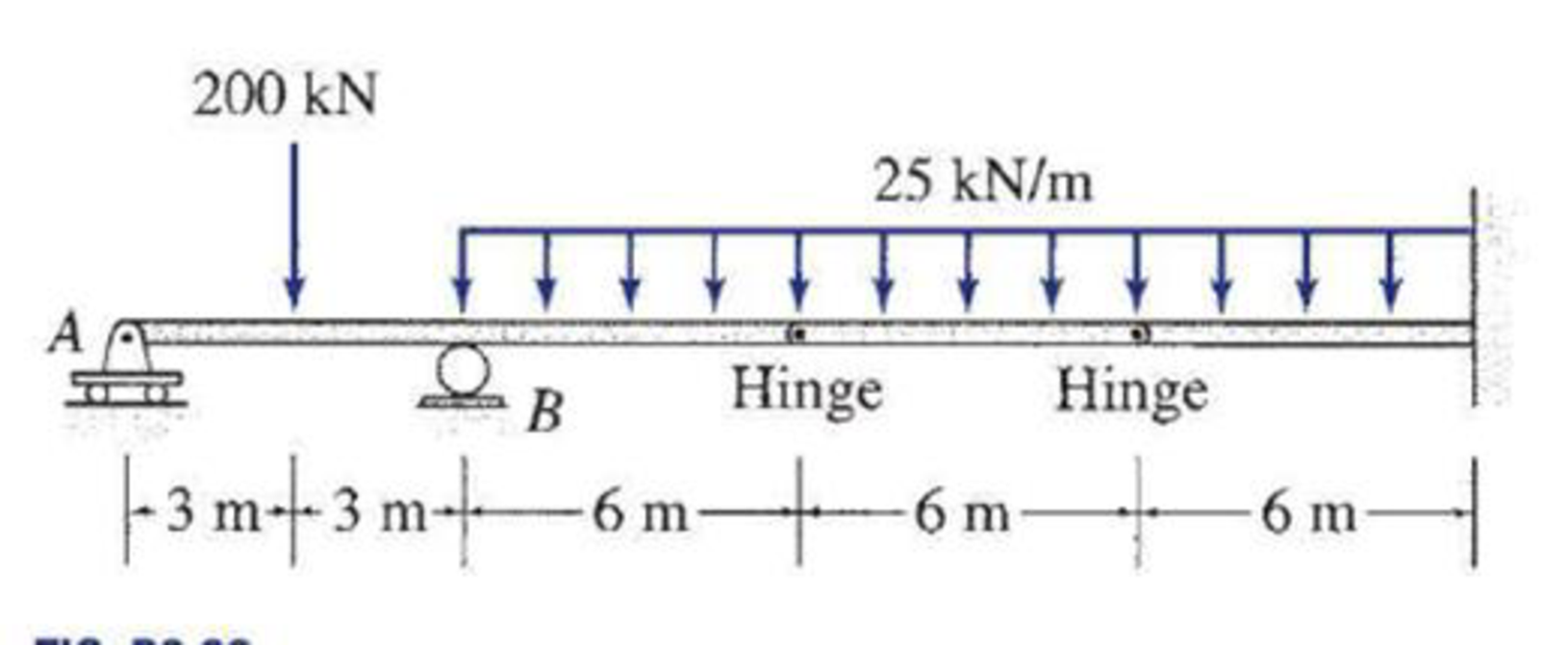# Determine the reactions at the supports for the structures shown. FIG.P3.39

#### Solutions

Chapter
Section
Chapter 3, Problem 39P
Textbook Problem
122 views

## Determine the reactions at the supports for the structures shown.FIG.P3.39

To determine

Calculate the support reactions for the given structure.

### Explanation of Solution

Given information:

The structure is given in the Figure.

Apply the sign conventions for calculating reaction forces and moments using the three equations of equilibrium as shown below.

• For summation of forces along x-direction is equal to zero (Fx=0), consider the forces acting towards right side as positive (+) and the forces acting towards left side as negative ().
• For summation of forces along y-direction is equal to zero (Fy=0), consider the upward force as positive (+) and the downward force as negative ().
• For summation of moment about a point is equal to zero (Matapoint=0), consider the clockwise moment as negative and the counter clockwise moment as positive.

Calculation:

Let Ay and By be the vertical reaction at the roller supports A and B.

Let Cx, Cy, and MC be the horizontal reaction, vertical reaction, and moment at the fixed support.

Sketch the free body diagram of the structure as shown in Figure 1.

Use equilibrium equations:

Summation of forces along x-direction is equal to 0.

+Fx=0Cx=0

Therefore, the horizontal reaction at A is Ax=0_.

For the member AD, the summation of moments about D is equal to 0.

For the member AE, the summation of moments about E is equal to 0.

MEAE=0Ay(18)By(12)+200(15)+25(12)(122)=018Ay12By=4,800 (2)

Solve Equation (1) and Equation (2) using calculator

### Still sussing out bartleby?

Check out a sample textbook solution.

See a sample solution

#### The Solution to Your Study Problems

Bartleby provides explanations to thousands of textbook problems written by our experts, many with advanced degrees!

Get Started

Find more solutions based on key concepts
For Problems 16.44 through 16.48, discuss how you would create the solid model of the given objects. See Exampl...

Engineering Fundamentals: An Introduction to Engineering (MindTap Course List)

What is meant by the term “shadow IT?

Fundamentals of Information Systems

Describe how a decision support system (DSS) is used. OLAP stands for _____.

Enhanced Discovering Computers 2017 (Shelly Cashman Series) (MindTap Course List)

What are the corresponding centerline and included angles to 5/8" TPF?

Precision Machining Technology (MindTap Course List)

What does the term 3 Vs refer to?

Database Systems: Design, Implementation, & Management

How can discontinuities in the root face be removed?

Welding: Principles and Applications (MindTap Course List)

If your motherboard supports ECC DDR3 memory, can you substitute non-ECC DDR3 memory?

A+ Guide to Hardware (Standalone Book) (MindTap Course List)